﻿ Stability Loss of Axisymmetric Cylindrical Shell under Axial Pressure LoadPublications are Open
Access in this journal
Article Versions
Export Article
• Normal Style
• MLA Style
• APA Style
• Chicago Style
Research Article
Open Access Peer-reviewed

### Stability Loss of Axisymmetric Cylindrical Shell under Axial Pressure Load

Tomáš Kula , Jozef Bocko, Róbert Huňady, Cosmin-Stefan Roszkos, Marek Schnitzer
American Journal of Mechanical Engineering. 2017, 5(6), 341-344. DOI: 10.12691/ajme-5-6-22
Published online: December 15, 2017

### Abstract

The paper deals with geometric imperfections, which are defined as the deviation of the mid-surface of the shell. A parametric model of the axially symmetrical shell was created which was loaded with axial compressive load. The task was to determine the decrease in critical force causing loss of stability. The finite element method was used in order to find solution.

### 1. Introduction

A group of thin-walled components is an inseparable part of construction solutions today. Their advantage is in relative high stiffness and carrying capacity in relation to their weight. For that reason they found use in areas such as aviation, mechanical engineering, structure and all areas where creation of oversized structures is a necessity. While creating a design, it is necessary to consider cases of stability loss that can occur as consequence of negative factors during operating load 1, 2.

One of the representatives of the thin-walled components is the axisymetric cylindrical shell. Stability loss of shells has its specifics, that differentiate them from the unstable bars or plates. Calculating critical load significantly differs from calculating critical modes of stability of geometrically simpler objects. Shells as such are very sensitive to irregularities of mid-surface. These shape irregularities significantly reduce the upper critical level. This also explains the large dispersion of results at experimental determination of critical levels 3.

We talk about shape stability loss when the conditions for an object to go from stable to labile equilibrium are met while this transition is characterized by change of object’s shape. Based on that we differentiate these states:

• stable equilibrium position,

• labile equilibrium position,

• indifferent equilibrium position 4.

### 2. Theory of Axisymetric Cylindrical Shell

Shell components are popular when constructing oversized parts. For example, different main storages that are used to store gaseous, liquid and solid substances. Based on paramaters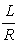(while L - shell lenght, R – shell radius) and it is possible to separate shells into the following groups:

a- very short cylinders,

b - moderately long cylinders,

c - long cylinders.

Each group is shows on Figure 1.

• Figure 1. Type of axisymetric cylindrical shell

Interestingly, each group of cylindrical shells has it’s own specifications that are significantly different. As an example we can take long cylinders that behave as Euler problem of compressed bar, when by load impact the cylindrical shell deflects to one side and assumes a new equilibrium configuration without damaging surface. On the other hand, the loss of stability of moderately long cylinders is caused by local stability losses which manifest as curl or they take on the shape of a diamond (so called diamond buckling). Such localized shape stability loss cause premature failure of the shell before the calculated level of critical load 5, 6, 7, 8.

### 3. Numerical Calculation of Cylindrical Shells Loss Stability

A static linear analysis is the basis for a general buckling problem and the equilibrium equation can be stated as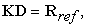(1)

where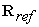is an arbitrary load on the structure,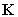is its stiffness matrix and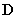is the displacement of nodal point. When the displacements are known, the stresses can be calculated for the used forces, which can be used to form the stress stiffness matrix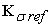. Since the stress stiffness matrixis proportional to the load vector, an arbitrary stress stiffness matrix and an arbitrary load vector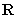may be defined by a constant as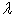.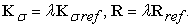(2)

The stiffness matrix is not changed by the applied load because the problem is treated linearly. A relation between the stiffness matrices, the displacement and the critical load can then be stated as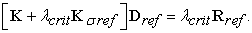(3)

The critical load is defined as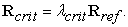(4)

Since the buckling is defined as a change in displacement with the same load, equation (3) is valid, where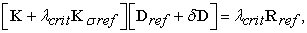(5)

where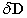is a buckling displacement increment. The point where a displacement is possible without a change in applied load, as in buckling, is called the bifurcation point. The difference between Eq. (3) and Eq. (5) gives an eigenvalue problem.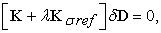(6)

where the smallest root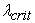defines the smallest load where there is a bifurcation. Trivial solutions of the eigenvalue problem are not of interest which means that the solution of Eq. 6, can be written as Eq. (7). However, several program have embedded solvers for eigenvalue problems so the last step is not always necessary.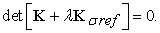(7)

The calculated eigenvalueis always connected to an eigenvector, which in a buckling problem is called a buckling mode. This buckling mode represents the shape of the structure after buckling has occurred. However, since the magnitude of is not determined in a linear buckling problem, the amplitude of the bucking mode is still unknown. It is important to understand that this theoretical solution of a linear buckling problem assumes that a perfect geometry is used and therefore the real critical load will be lower than the calculated critical load if an imperfection is present. A linear buckling calculation, hence, gives an upper limit of the buckling load for a specific geometry. 9 and 10.

### 4. Definition of Geometric Imperfections and Creating Variants

Geometrical imperfection is defined by two parameters. First parameter causes narrowing (decrease) of the nominal diameter of the shell, labeled as e. Second parameter, labeled as el, defines the length of the narrowed portion of the shell.

The parametric model (Figure 2) on which the calculations were made was created in the MATLAB program. To perform the calculations themselves, ABAQUS software was used.

• Figure 2. Created a half model of cylindrical shell with imperfection

In first variant, the length of the narrowing on the shell is defined as el1 = 500 mm, in second variant it is el2 = 250 mm and in thirs variant it is el3 = 125 mm, while the total length of the cylindrical shell is L = 1000 and nominal radius is R = 100 mm. For a more accurate comparison of the result, the thickness of the shell’s wall was variable and its value varied between 1 mm and 10 mm.

• Figure 3. Mesh and defined boundary condition

For the numerical calculations, the boundary conditions were defined so that they approximate as closely as possible the real condition that may occur. It is assumed that one end is fixed and on the second, free end, there is a load defined as on Figure 3

### 5. Results

A total of 210 calculations were done. Based on the results, a graph (chart) was constructed between each parameter (Figure 4 - Figure 6).

• Figure 4. Chart of critical force Fcr
• Figure 5. Chart of critical force Fcr
• Figure 6. Chart of critical force

The model with which it was worked was designed as the third group of shells, long shell. For this reason, it was assumed that the calculated own shapes would be similar to Euler's problem of pressed bar. One of the custom shapes is shown in Figure 7.

• Figure 7. One of the buckling mode shape

Graphs depicting the drop of selected forces are shown below.

• Figure 8. Difference between critical forces
• Figure 9. Difference between critical forces
• Figure 10. Difference between critical forces

Black line present the reference critical force of axisymmetric cylindrical shell without geometrical imperfection eref = 0. Red line present the critical force of axisymetric cylindrical shell with geometrical imperfection, where value of e is e1 = 15 mm and green line present the critical force for same case, but with e value e2 = 30 mm. Some text is for Figure 9 and Figure 10.

### 6. Conclusion

Based on the presented results, it can be generally confirmed that the geometric deviation from the mid-surface has a negative effect on the final strength of the shell. By considering the results obtained for each variant of the imperfections, the following conclusions can be made. One of the major negative effects for the resulting shell strength is the length of imperfection. It can be observed that if the length of the imperfection is small compared to the length of the shell (when the damaged site is 12.5% of the total length), the critical force values decrease considerably, compared to when the length of the imperfection is greater (when the narrowing of the shell is 50% of the total length). Thus, it can be argued that circular design construction components, whose lengths of damaged places are considerably shorter than their length Figure 7 collapse under the impact of load sooner than components whose length of damaged places is larger and the damage continuously passes from undamaged part of the shell to the part affected by the imperfection (first variant).

One of the other negative factors that were observed and significantly influenced the strength of the shell was the change in the nominal size of the shell. The larger the narrowing of the shell was, the more markedly the values of critical force Fcr dropped, which can be seen in Figure 8 - Figure 10. Thus again it is possible to conclude that that the greater the narrowing of the shell diameter becomes, the sooner the constructional component of rotational character collapses.

### Acknowledgements

Results presented in this work were obtained with the support of the national agency’s grant VEGA 1/0731/16 “Development of modern numerical and experimental methods of analysis of mechanical systems”.

### References

  Trebuňa, F., Šimčák, F.: Tenkostenné nosné prvky a konštrukcie. Košice: TU, 1999. 268 s. In article  Simitses, G.J., Hodges, D.H.: Fundamentals of Structural Stability. Elsevier Inc., 2006. 389 p. In article View Article  Trebuňa, F., Šimčák, F.: Pružnosť a pevnosť pre odbory: Výrobné technológie. In article  Trebuňa, F., Šimčák, F. Odolnosť prvkov mechanických sústav, Emilena, Košice, 2004, 911-940. In article  Narayanan, R.: Shell Structures, Stability and Strength. Elsevier, 1985. 345 p. In article  Donnell, H.L.: Beams, Plates and Shells. USA: McGraw-Hill, Inc. 1976. 453 p. In article PubMed  Chen, D.H.: Crush Mechanics of Thin-Walled Tubes. USA: CRC Press, 2016. 329 p. In article View Article  Iványi, M., Škaloud, M.: Stability Problems of Steel Structures. Italy: Springer-Verlag, 1992. 415 p. In article View Article  Wallin, M.: A Finite Element Tool for Linear Buckling Analysis. Linköping University Institute of Technology, 2014. 40 p. Master thesis. In article View Article  Frank, A.L., Larsen, K.S.: Methods for Decreasing the Total Solution Time of Linear Buckling Finite Element Analyses. Aalborg University, 2012. 78 p. Master thesis. In article View ArticleThis work is licensed under a Creative Commons Attribution 4.0 International License. To view a copy of this license, visit http://creativecommons.org/licenses/by/4.0/